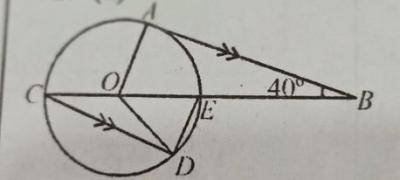### 2020 WAEC Mathematics Theory (a) In the diagram, AB is a tangent to the circle with centre O, and COB...

WAEC 2020(a) In the diagram, AB is a tangent to the circle with centre O, and COB is a straight line. If CD//AB and < ABE = 40°, find: < ODE.

(b) ABCD is a parallelogram in which |$$\overline{CD}$$| = 7 cm, I$$\overline{AD}$$I = 5 cm and < ADC= 125°.

(i) Illustrate the information in a diagram.

(ii) Find, correct to one decimal place, the area of the parallelogram.

(c) If x = $$\frac{1}{2}$$(1 - $$\sqrt{2}$$). Evaluate (2x$$^2$$ - 2x).

##### Explanation

< AOB = 108$$^o$$ - (90$$^o$$ + 40$$^o$$)

= 180$$^o$$ - 130$$^o$$ = 50$$^o$$

< DCO = 40$$^o$$ (Alternate angle)

< ODE = $$\frac{180^o - 80^o}{2}$$ = $$\frac{100^o}{2}$$

= 50$$^o$$

(b)(i)(b)(ii) Area of the parallelogram

= ab sin $$\theta$$

= 5cm x 7cm sin 125$$^3$$

= 35 sin 125

= 28.7cm$$^2$$

(c)

x = $$\frac{1}{2}(1 - \sqrt{2})$$

evaluate (2x$$^2$$ - 2x)

= 2[$$\frac{1}{2}(1 - \sqrt{2})^2$$] - 2[$$\frac{1}{2} (1 - \sqrt{2}$$)]

= 2[$$\frac{1}{4}(1 - 2\sqrt{2} + 2)$$] - 1 + $$\sqrt{2}$$

= 2[$$\frac{1}{4}(3 - 2\sqrt{2})$$ - 1 + $$\sqrt{2}$$]

2[$$\frac{3}{4} - \frac{1}{2} \sqrt{2} - 1 + \sqrt{2}$$]

= $$\frac{3}{2} - \sqrt{2} - 2 + 2\sqrt{2}$$

= $$\frac{1}{2} + \sqrt{2}$$

#### Contributions ({{ comment_count }})

{{ settings.no_comment_msg ? settings.no_comment_msg : 'There are no comments' }}

#### Quick Questions

• 2 Answers   ·   46 minutes ago
• 3 Answers   ·   50 minutes ago
• 2 Answers   ·   Mathematics   ·   2 hours ago